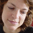Processing.js

An image is recreated from its individual component colors. The many colors of the image are created through modulating the red, green, and blue values. This is an exageration of an LCD display.// All Examples Written by Casey Reas and Ben Fry
// unless otherwise stated.
size(200, 200);
noStroke();
background(0);

// Load an image from the data directory
PImage c;

int xoff = 0;
int yoff = 0;
int p = 2;
int pix = p*3;

for(int i = 0; i < c.width*c.height; i += 1)
{
int here = c.pixels[i];

fill(red(here), 0, 0);
rect(xoff, yoff, p, pix);

fill(0, green(here), 0);
rect(xoff+p, yoff, p, pix);

fill(0, 0, blue(here));
rect(xoff+p*2, yoff, p, pix);

xoff+=pix;
if(xoff >= width-pix) {
xoff = 0;
yoff += pix;
}
}